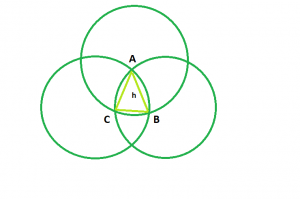# Area of Reuleaux Triangle

• Last Updated : 17 Mar, 2021

Given an integer h which is the side of an equilateral triangle formed by the same vertices as the Reuleaux triangle, the task is to find and print the area of the Reuleaux triangle.Examples:

Input: h = 6
Output: 25.3717
Input: h = 9
Output: 57.0864

Approach: The shape made by the intersection of the three circles ABC is the Reuleaux Triangle and the triangle formed by the same vertices i.e. ABC is an equilateral triangle with side h

Now, Area of sector ACB, A1 = (π * h2) / 6
Similarly, Area of sector CBA, A2 = (π * h2) / 6
And, Area of sector BAC, A3 = (π * h2) / 6
So, A1 + A2 + A3 = (π * h2) / 2
Since, the area of the triangle is added thrice in the sum.
So, Area of the Reuleaux Triangle, A = (π * h2) / 2 – 2 * (Area of equilateral triangle) = (π – √3) * h2 / 2 = 0.70477 * h2

Below is the implementation of the above approach:

## C++

 `// C++ Program to find the area``// of Reuleaux triangle``#include ``using` `namespace` `std;` `// Function to find the Area``// of the Reuleaux triangle``float` `ReuleauxArea(``float` `a)``{` `    ``// Side cannot be negative``    ``if` `(a < 0)``        ``return` `-1;` `    ``// Area of the Reuleaux triangle``    ``float` `A = 0.70477 * ``pow``(a, 2);``    ``return` `A;``}` `// Driver code``int` `main()``{``    ``float` `a = 6;``    ``cout << ReuleauxArea(a) << endl;``    ``return` `0;``}`

## Java

 `// Java Program to find the area``// of Reuleaux triangle` `public` `class` `GFG``{``    ``// Function to find the Area``    ``// of the Reuleaux triangle``    ``static` `double` `ReuleauxArea(``float` `a)``    ``{``    ` `        ``// Side cannot be negative``        ``if` `(a < ``0``)``            ``return` `-``1``;``    ` `        ``// Area of the Reuleaux triangle``        ``double` `A = (``double``)``0.70477` `* Math.pow(a, ``2``);``        ``return` `A;``    ``}``    ` `    ``// Driver code``    ``public` `static` `void` `main(String args[])``    ``{``        ``float` `a = ``6``;``        ``System.out.println(ReuleauxArea(a)) ;``    ``}``    ``// This code is contributed by Ryuga``}`

## Python3

 `# Python3 program to find the area``# of Reuleaux triangle``import` `math as mt` `# function to the area of the``# Reuleaux triangle``def` `ReuleauxArea(a):``    ` `    ``# Side cannot be negative``    ``if` `a < ``0``:``        ``return` `-``1``    ``# Area of the Reauleax triangle``    ``return` `0.70477` `*` `pow``(a, ``2``)` `# Driver code``a ``=` `6``print``(ReuleauxArea(a))` `# This code is contributed``# by Mohit Kumar       `

## C#

 `// C# Program to find the area ``// of Reuleaux triangle ``  ` `using` `System;` `public` `class` `GFG``{``    ``// Function to find the Area ``    ``// of the Reuleaux triangle ``    ``static` `double` `ReuleauxArea(``float` `a) ``    ``{ ``      ` `        ``// Side cannot be negative ``        ``if` `(a < 0) ``            ``return` `-1; ``      ` `        ``// Area of the Reuleaux triangle ``        ``double` `A = (``double``)0.70477 * Math.Pow(a, 2); ``        ``return` `A; ``    ``} ``      ` `    ``// Driver code ``    ``public` `static` `void` `Main() ``    ``{ ``        ``float` `a = 6; ``        ``Console.WriteLine(ReuleauxArea(a)) ; ``    ``}``}``    ``// This code is contributed by Subhadeep`

## PHP

 `

## Javascript

 ``
Output:
`25.3717`

My Personal Notes arrow_drop_up